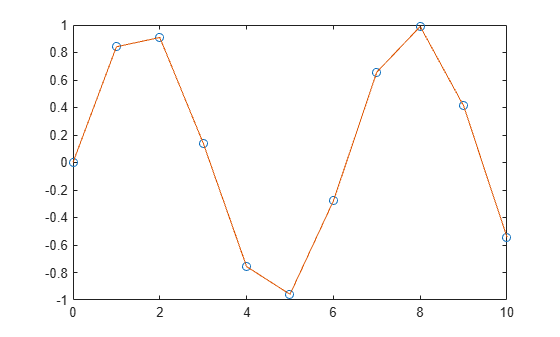# interp1q

(Not recommended) Quick 1-D interpolation

`interp1q` is not recommended. Use `interp1` instead.

## Syntax

``yi = interp1q(x,Y,xi)``

## Description

example

````yi = interp1q(x,Y,xi)` returns the interpolated value of the 1-D function `Y` at the points of column vector `xi`. The vector `x` specifies the coordinates of the underlying interval.```

## Examples

collapse all

Generate a coarse sine curve and interpolate over a finer abscissa.

```x = (0:10)'; y = sin(x); xi = (0:.25:10)'; yi = interp1q(x,y,xi); plot(x,y,'o',xi,yi)```## Input Arguments

collapse all

Sample points, specified as a monotonically increasing column vector. The sample points in `x` are the x-coordinates of the sample data in `Y`.

Example: `[1; 2; 3; 4]`

Example: `[1.1; 2.4; 3.2; 4.7]`

Data Types: `single` | `double`
Complex Number Support: Yes

Sample data, specified as a column vector or matrix. The sample data in `Y` represents function values at the coordinates in `x`.

• If `Y` is a column vector, then it must have `length(x)` elements. The length of output `yi` is equal to `length(xi)`.

• If `Y` is a matrix, then it must have `length(x)` rows and the interpolation is performed for each column of `Y`. In that case, the size of `yi` is `length(xi)`-by-`size(Y,2)`.

Example: `[1; 4; 9; 16]`

Example: `[0.1; 1.4; 2.2; 3.7]`

Data Types: `single` | `double`
Complex Number Support: Yes

Query points, specified as a column vector. The query points are locations where `interp1q` uses linear interpolation to determine the approximate value of the function represented by `Y`.

`interp1q` returns `NaN` for any values of `xi` that lie outside the coordinates in `x`.

Example: `[1.5; 2.5]`

Data Types: `single` | `double`
Complex Number Support: Yes

## Version History

Introduced before R2006a